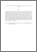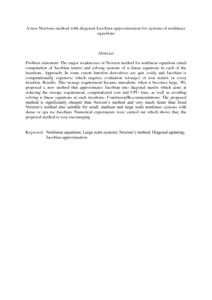# A new Newtons method with diagonal Jacobian approximation for systems of nonlinear equations

## Citation

Yusuf, Mohammed Waziri and Leong, Wah June and Abu Hassan, Malik and Monsi, Mansor (2010) A new Newtons method with diagonal Jacobian approximation for systems of nonlinear equations. Journal of Mathematics and Statistics, 6 (3). pp. 246-252. ISSN 1549-3644; ESSN: 1558-6359

## Abstract

Problem statement: The major weaknesses of Newton method for nonlinear equations entail computation of Jacobian matrix and solving systems of n linear equations in each of the iterations. Approach: In some extent function derivatives are quit costly and Jacobian is computationally expensive which requires evaluation (storage) of n×n matrix in every iteration. Results: This storage requirement became unrealistic when n becomes large. We proposed a new method that approximates Jacobian into diagonal matrix which aims at reducing the storage requirement, computational cost and CPU time, as well as avoiding solving n linear equations in each iterations. Conclusion/Recommendations: The proposed method is significantly cheaper than Newton’s method and very much faster than fixed Newton’s method also suitable for small, medium and large scale nonlinear systems with dense or spa rse Jacobian. Numerical experiments were carried out which shows that, the proposed method is very encouraging.Preview
PDF (Abstract)
A new Newtons method with diagonal Jacobian approximation for systems of nonlinear equations.pdfView Item